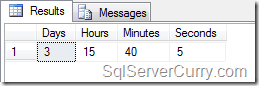### Date Difference in SQL Server in Days, Hours, Minutes and Seconds

A lot of users often ask questions related to finding difference between two date. The results are expected in Days, Hours, Minute and Seconds. Here’s a query that shows how to find the difference between two dates in SQL Server

Here’s the query in a readable format to try out

DECLARE @Startdate DATETIME, @Enddate DATETIME
SET @Startdate = '2011-01-02 11:35:26'
SET @Enddate = '2011-01-06 03:15:31'

-- Query by SqlServerCurry.com
-- Total seconds in a day
DECLARE @TotalSec int
SET @TotalSec = 24*60*60;

-- Convert DateDiff into seconds
DECLARE @DiffSecs int
SET @DiffSecs = DATEDIFF(SECOND, @Startdate, @Enddate)

SELECT
CONVERT(char(2), (@DiffSecs/@TotalSec))as [Days],
CONVERT(char(2), ((@DiffSecs%@TotalSec)/3600)) as [Hours],
CONVERT(char(2), (((@DiffSecs%@TotalSec)%3600)/60)) as [Minutes],
CONVERT(char(2), (((@DiffSecs%@TotalSec)%3600)%60)) as [Seconds]

The query shown above is quite simple. We first calculate the total seconds in a day (86400) and store it in a variable. We then use the DATEDIFF function and calculate the difference between two dates in seconds and store it in another variable. Finally we use the CONVERT function to apply some calculations and get the difference between two dates in days, hours, minutes and seconds

OUTPUT